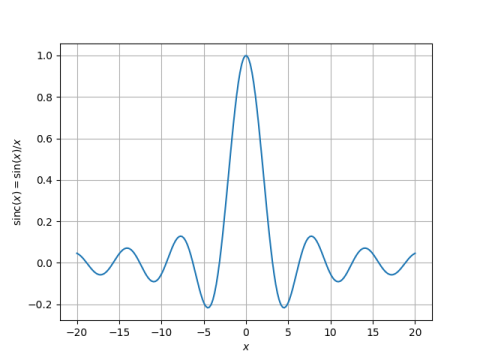# Rate of convergence for Newton’s method

In the previous post I looked at the problem of finding the peaks of the sinc function.In this post we use this problem to illustrate how two formulations of the same problem can behave very differently with Newton’s method.

The previous post mentioned finding the peaks by solving either

x cos x – sin x = 0

or equivalently

tan xx = 0

It turns out that the former is much better suited to Newton’s method. Newton’s method applied to the first equation will converge quickly without needing to start particularly close to the root. Newton’s method applied to the second equation will fail to converge at all unless the method beings close to the root, and even then the method may not be accurate.

Here’s why. The rate of convergence in solving

f(x) = 0

with Newton’s method is determined by the ratio of the second derivative to the first derivative

| f ‘ ‘ (x) / f ‘ (x) |

near the root.

Think of the second derivative as curvature. Dividing by the first derivative normalizes the scale. So convergence is fast when the curvature relative to the scale is small. Which makes sense intuitively: When a function is fairly straight, Newton’s method zooms down to the root. When a function is more curved, Newton’s method requires more steps.

The following table gives the absolute value of the ratio of the second derivative to the first derivative at the first ten peaks, using both equations. The bound on the error in Newton’s method is proportional to this ratio.

```    |------+------------+------------|
| peak | Equation 1 | Equation 2 |
|------+------------+------------|
|    1 |      0.259 |       15.7 |
|    2 |      0.142 |       28.3 |
|    3 |      0.098 |       40.8 |
|    4 |      0.075 |       53.4 |
|    5 |      0.061 |       66.0 |
|    6 |      0.051 |       78.5 |
|    7 |      0.044 |       91.1 |
|    8 |      0.039 |      103.7 |
|    9 |      0.034 |      116.2 |
|   10 |      0.031 |      128.8 |
|------+------------+------------|
```

The error terms are all small for the first equation, and they get smaller as we look at peaks further from the origin. The error terms for the second equation are all large, and get larger as we look at peaks further out.

For the third and final post in this series, see Peaks of Sinc.